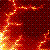Discussion about math, puzzles, games and fun.   Useful symbols: ÷ × ½ √ ∞ ≠ ≤ ≥ ≈ ⇒ ± ∈ Δ θ ∴ ∑ ∫  π  -¹ ² ³ °

You are not logged in.

## #1 2007-10-24 07:55:50

bossk171
MemberRegistered: 2007-07-16
Posts: 305

### Mathematical Hyperspace

I read this book called, "An Imaginary Tale: The Story of √-1" by Paul J. Nahin. It's pretty good, but there's one part in the book that I got kind of hung up on.

In this post, "x" will always be a real number.

He shows that by using the differential arc length formula:

And by using the distance formula:

That for any real curve y, you can find the ratio of the distance of the arc length to the distance of the secant, obviously that ratio will always be more than 1 because the shortest distance between to points is a strait line.

But then he uses the complex function, y(x) = x² + ix and shows that the ratio is less than one. Personally I think that real cool.

The issue I'm having though, is that I think his equations are wrong. If I were to graph y(x) = x² + ix I would graph it so that x was the domain, y was the real part of the range, and z was the imaginary part of the range (making the y,z plane the complex plane). But then the arc length would be a different formula altogether, and the ratio would we greater than 1 (like it is for functions whose domain and range are entirely real).

Is my reasoning way off? What would be the arc length formula?

There are 10 types of people in the world, those who understand binary, those who don't, and those who can use induction.

Offline